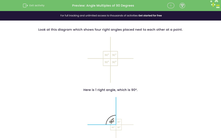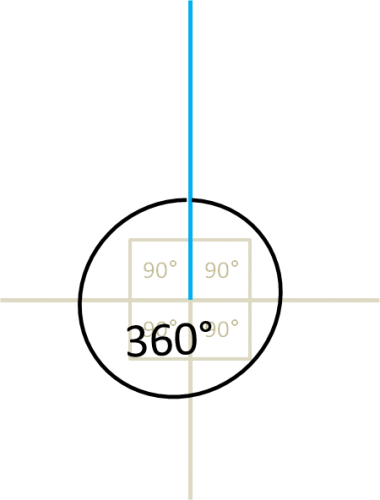# Angle Multiples of 90 Degrees

In this worksheet, students must identify angles which are multiples of 90° by counting right angles.Key stage:  KS 2

Curriculum topic:   Geometry: Properties of Shapes

Curriculum subtopic:   Identify Angles at a Point

Popular topics:   Angles worksheets

Difficulty level:#### Worksheet Overview

Look at this diagram which shows four right angles placed next to each other at a point.Here is 1 right angle, which is 90°.Here are 2 right angles put together, giving an angle of 180°.

2 × 90° = 180°Here are 3 right angles put together, giving an angle of 270°.

3 × 90° = 270°Here are 4 right angles put together, giving an angle of 360°.

4 × 90° = 360°### What is EdPlace?

We're your National Curriculum aligned online education content provider helping each child succeed in English, maths and science from year 1 to GCSE. With an EdPlace account you’ll be able to track and measure progress, helping each child achieve their best. We build confidence and attainment by personalising each child’s learning at a level that suits them.

Get started